## GPU Gems 3

The CD content, including demos and content, is available on the web and for download.

You can also subscribe to our Developer News Feed to get notifications of new material on the site.

# Chapter 32. Broad-Phase Collision Detection with CUDA

Scott Le Grand
NVIDIA Corporation

Collision detection among many 3D objects is an important component of physics simulation, computer-aided design, molecular modeling, and other applications. Most efficient implementations use a two-phase approach: a broad phase followed by a narrow phase. In the broad phase, collision tests are conservative—usually based on bounding volumes only—but fast in order to quickly prune away pairs of objects that do not collide with each other. The output of the broad phase is the potentially colliding set of pairs of objects. In the narrow phase, collision tests are exact—they usually compute exact contact points and normals—thus much more costly, but performed for only the pairs of the potentially colliding set. Such an approach is very suitable when a lot of the objects are actually not colliding—as is the case in practice—so that many pairs of objects are rejected during the broad phase.

In this chapter, we present a GPU implementation of broad-phase collision detection ("broad phase," for short) based on CUDA that is an order of magnitude faster than the CPU implementation.

The brute-force implementation of broad phase for n objects consists in conducting n(n-1)/2 collision tests. Thus it has a complexity of O(n 2). Alternative algorithms, such as sort and sweep(described in Section 32.1.1) or spatial subdivision (described in Section 32.1.2), achieve an average complexity of O(n log n) (their worst-case complexity remains O(n 2)). In Section 32.1.3, we outline the complications arising from a parallel implementation of spatial subdivision and how we deal with them. Our CUDA implementation of parallel spatial subdivision is described in Section 32.2.

#### 32.1.1 Sort and Sweep

One approach to the broad phase, as mentioned, is the sort and sweep algorithm (Witkin and Baraff 1997). In this approach, the bounding volume of each object i is projected onto the x, y, or z axis, defining the one-dimensional collision interval [bi , ei ] of the object along this axis; bi marks the beginning of the collision interval, and ei marks its end. Two objects whose collision intervals do not overlap cannot possibly collide with each other, leading to the algorithm that follows. The two markers of each object are inserted into a list with 2n entries (two markers for each object). Next, this list is sorted in ascending order. Finally, the list is traversed from beginning to end. Whenever a bi marker is found in the list, object i is added to a list of active objects. Whenever an ei marker is found, object i is removed from the list of active objects. Collision tests for object i are performed only between object i and all objects currently in the list of active objects at the moment when object i itself is added to this list of active objects. An example with three objects is illustrated in Figure 32-1.

Sort and sweep is simple to implement, and it is a great starting point for a broad-phase collision detection algorithm. Additionally, due to spatial coherency between one frame of simulation and the next, one can often use an O(n 2) sort such as insertion sort in an efficient manner to accelerate the algorithm, because of its improved O(n) performance when applied to mostly sorted lists.

#### 32.1.2 Spatial Subdivision

Spatial subdivision is an alternative to sort and sweep. Spatial subdivision partitions space into a uniform grid, such that a grid cell is at least as large as the largest object. Each cell contains a list of each object whose centroid is within that cell. A collision test between two objects is then performed only if they belong to the same cell or to two adjacent cells. Alternatively—and this is the approach taken in the rest of the chapter—one can assign to each cell the list of all objects whose bounding volume intersects the cell. In this case, an object can appear in up to 2 d cells, where d is the dimension of the spatial subdivision (for example, d = 3 for a 3D world), as illustrated in Figure 32-2.

A collision test between two objects is performed only if they appear in the same cell and at least one of them has its centroid in this cell. For example, in Figure 32-3, a collision test is performed between objects o 1 and o 2 because both have their centroids in cell 1, and between objects o 2 and o 3 because both appear in cell 5 and o 3 has its centroid in cell 5. But no collision test is performed between o 2 and o 4 because although both appear in cell 2, neither of their centroids is in cell 2.

The simplest implementation of spatial subdivision creates a list of object IDs along with a hashing of the cell IDs in which they reside, sorts this list by cell ID, and then traverses swaths of identical cell IDs, performing collision tests between all objects that share the same cell ID.

The necessity for a cell to be at least as big as the bounding volume of the largest object can cause unnecessary computation where there is great disparity in size between all of the objects. One such example would be a large planet surrounded by thousands of much smaller asteroids. Hierarchical grids (Mirtich 1996) address this issue, but they are beyond the scope of this chapter.

#### 32.1.3 Parallel Spatial Subdivision

Parallelizing spatial subdivision complicates the algorithm a bit. The first complication arises from the fact that a single object can be involved in more than one collision test simultaneously if it overlaps multiple cells and these cells are processed in parallel. Therefore, some mechanism must exist to prevent two or more computational threads from updating the state of the same object at the same time. To deal with this issue, we exploit the spatial separation of the cells. Because each cell is guaranteed to be at least as large as the bounding volume of the largest object in a computation, then it is guaranteed that as long as each cell processed during a computational pass is separated from every other cell being processed at the same time by at least one intervening cell, then only one cell containing each object will be updated at once. In 2D, this means that there must be four computational passes to cover all possible cells, which is illustrated in Figure 32-4: all cells with the same numeric label (1 to 4), or cell type, can be processed in the same pass. This figure illustrates a simple case wherein object 2 has two potential collisions that must be processed separately. In 3D, eight passes are required as the repeating unit in 2D changes from a repeating 2x2 square of cells numbered 1 to 4 to a repeating 2x2x2 cube of cells numbered 1 to 8, as illustrated in Figure 32-5.

There are two special cases that modify the need for ordering cell traversal. First, in the event that we are not changing the state of the objects but only performing collision tests, these 2 d passes can be collapsed into a single pass. And second, in the event that we are using this collision system to perform Jacobi versus Gauss-Seidel physics integration (Erleben et al. 2005), we can also handle all cells in one pass. In that case, though, there is the caveat that each object has 2 d velocity impulse outputs (one corresponding to each of the 2 d collision cells it could occupy) that are summed together for each object after collision processing is complete.

Another complication arising from parallel execution is that we must avoid performing the same collision test between two objects multiple times. This can happen if the two objects occupy an overlapping set of cells and their centroids are not in the same cell.

We deal with this possibility by doing the following:

1. Associating to each object a set of d + 2 d control bits; d bits hold the type of the cell where its centroid resides, or its home cell; 2 d bits specify the types of the cells intersected by its bounding volume.
2. Scaling the bounding sphere of each object by sqrt(2) and ensuring that the grid cell is at least 1.5 times as large as the scaled bounding sphere of the largest object.

Before performing a collision test between two objects during pass T, we first find out if one of the objects' home cell type, say T', is both less than T and among the cell types that are common to both objects (obtained by ANDing their 2 d control bits). If this is the case, we can skip the test because either the objects share a cell of type T', in which case the test has already been performed during pass T'; or they do not share a cell of type T', in which case their bounding volumes cannot possibly overlap because of point 2 we just mentioned. The first case is illustrated by objects o 1 and o 2 in Figure 32-6. The test is skipped in pass T = 3 because both objects share cell types 1 and 3, and o 2's home cell type is T'= 1. Skipping the test is indeed safe because it has already been performed in pass 1. The second case is illustrated by objects o 3 and o 4 in Figure 32-6. The test is skipped in pass T = 4 because both objects share cell types 3 and 4, and o 4's home cell type is T'= 3. Skipping the test is indeed safe because both objects cannot possibly collide: They overlap different cells of type 3, so their centroids are separated by a distance greater than the diameter of the largest object.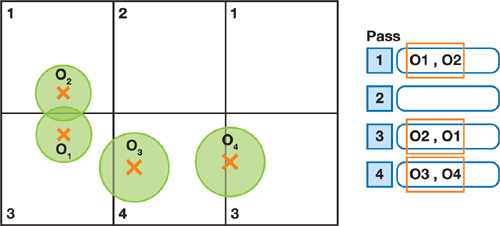Figure 32-6 Control Bits and Large Enough Cells Allow Us to Avoid Performing the Same Collision Test Between Two Objects Multiple Times

## 32.2 A CUDA Implementation of Spatial Subdivision

In this section, we provide the details of our implementation using CUDA. In Section 32.2.1, we describe how we store the list of object IDs and cell IDs in device memory. In Section 32.2.2, we show how we build the list of cell IDs from the object's bounding boxes. In Section 32.2.3, we provide an in-depth description of the algorithm we use to sort the list. In Section 32.2.4, we show how we build an index table so that we can traverse this sorted list, as outlined in Section 32.2.5, and schedule pairs of objects for morerigorous collision detection during the narrow phase. All the figures illustrating the whole process throughout this section correspond to the example in Figures 32-7 and 32-8.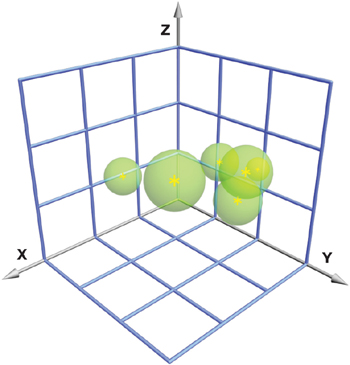Figure 32-7 A Set of Objects Superimposed on the Uniform Grid That Partitions 3D Space

#### 32.2.1 Initialization

The attributes of each object are its ID (which is used to access additional object properties such as its bounding volume during low-level collision tests and construction of the cell ID array discussed next), its control bits (used to store various bit states, such as the home cell type and other occupied cell types, as mentioned at the end of Section 32.1.2), and up to 2 d IDs of the cells covered by its bounding volume. The most natural way of storing these is as an array of structures. To reduce bandwidth requirements when sorting this array, though, it is preferable to store these attributes in two separate arrays of (n x 2 d ) elements each:

• The first array, the cell ID array, contains the cell IDs of the first object followed by the cell IDs of the second object, and so on.
• The second array, the object ID array, contains the object IDs and control bits for each object, once for each cell it occupies.

It is implicitly assumed in the rest of the description of the algorithm that each time the elements of the cell ID array are reordered, the elements of the object ID array are reordered the same way.

#### 32.2.2 Constructing the Cell ID Array

Once sufficient storage has been allocated, the first step in performing collision detection is to populate the cell ID array. For a given object, there may be cells that overlap with its bounding volume but are not its home cell, or H cell. We call such overlapping cells the phantom cells, or P cells, of that object. As indicated earlier, if a cell is a P cell for one object and a P cell for another object, then these objects can never collide in this cell because the centroids of the two objects in question are separated by at least one intervening cell, and by design, the cell diameter is at least twice the radius of the largest object.

The H cell ID of each object is simply a hash of its centroid's coordinates. A typical 3D hash would resemble this:

`    GLuint hash = ((GLuint)(pos.x / CELLSIZE) << XSHIFT) |               ((GLuint)(pos.y / CELLSIZE) << YSHIFT) |               ((GLuint)(pos.z / CELLSIZE) << ZSHIFT); `

Here, pos represents the position of the object; CELLSIZE is the dimension of the cells; and XSHIFT, YSHIFT, and ZSHIFT are predefined constants determining how many bits are assigned to the hash of each dimension's coordinate. This hash is stored in the cell ID array as the first cell ID for each object. Simultaneously, the corresponding element in the object ID array is updated with the object ID, and we set a control bit to indicate that this is the object's H cell.

Next, the P cell IDs of each object are stored in the cell ID array by testing to see if its bounding volume overlaps any of its H cell's 3 d - 1 immediate neighbors. Given that the cell size is at least as large as the largest bounding volume, an object can touch only 2d cells at once. This means that an object has one H cell and that it can have up to 2d - 1 P cells. If the object has fewer than 2 d - 1 P cells, the extra cell IDs are marked as 0xffffffff to indicate they are not valid.

The creation of these H and P cell IDs is performed for each object by one thread. To accommodate for the case in which there are more objects than threads, each thread handles multiple objects. More precisely, thread j of thread block i handles objects iB + j, iB + j + nT, iB + j + 2nT, and so on, where B is the number of threads per block and T is the total number of threads.

Figure 32-9 illustrates the result of cell ID array construction.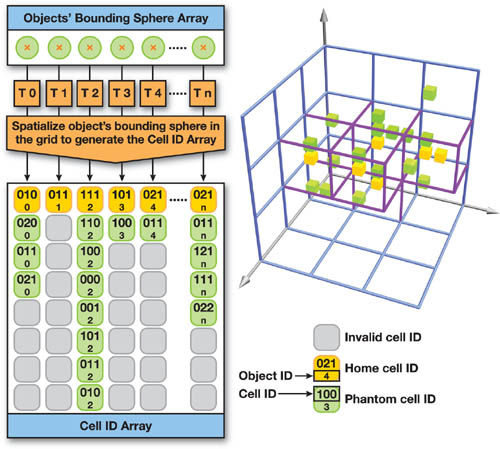Figure 32-9 Initial Contents of the Cell ID Array for the Objects of After Construction

After all cell IDs have been created, we need to count them to compute their total number for use during the creation of the collision cell list in Section 32.2.4. The most efficient way to add multiple values together on a parallel machine is through a parallel reduction. In CUDA, the threads of a thread block can cooperate through shared memory, yielding a very fast implementation of parallel reduction. (See Chapter 39 of this book, "Parallel Prefix Sum (Scan) with CUDA," for all the details.) Therefore, we first calculate the number of cell IDs created by each thread block. This count is written out for each block to global memory. Once all blocks have finished this process, a single block is launched to add the results of the preceding blocks.

#### 32.2.3 Sorting the Cell ID Array

Next, the cell ID array is sorted by cell ID. Of course, each cell ID appears multiple times in the array in general—each time there is more than one bounding volume that intersects a cell. We want elements with the same cell ID to be sorted by cell ID type (H before P), because this simplifies the collision cell processing of Section 32.2.5, where we iterate over all H cell IDs against the total of all H and P cell IDs. Because we have already ordered the cell ID array first by object ID and second by cell ID type (H or P), the choice of sort algorithm is therefore limited to a stable sort. A stable sort guarantees that at the end of the sorting process, identical cell IDs remain in the same order that they were in at the beginning of it. Figure 32-10 illustrates the sorting process applied to the cell ID array of Figure 32-9.

We first outline the radix sort algorithm and then describe our parallel implementation in detail.

A radix sort sorts keys—in our case, the 32-bit cell IDs—with B bits by groups of L bits within them—where L < B— in as many successive passes as there are groups (Sedgewick 1992). For example, a common radix sort on 32-bit keys would set L to 8, resulting in a four-pass radix sort, because B/L is equal to 4 in that case.

The most surprising feature of a radix sort is that it sorts low-order bits before higherorder bits. The aforementioned stability of a radix sort guarantees that this unexpected direction of sorting works; that is, keys already sorted by their lowest 8 bits will not lose this ordering when the keys are subsequently sorted by higher-order bits during future passes.

Each sorting pass of a radix sort proceeds by masking off all bits of each key except for the currently active set of L bits, and then it tabulates the number of occurrences of each of the 2 L possible values that result from this masking. The resulting array of radix counters is then processed to convert each value into offsets from the beginning of the array. In other words, each value of the table is replaced by the sum of all its preceding values; this operation is called a prefix sum. Finally, these offsets are used to determine the new positions of the keys for this pass, and the array of keys is reordered accordingly.

#### The Parallel Radix Sort Algorithm

Each pass takes a cell ID array as input and outputs the reordered elements to another cell ID array. The input array for the first pass is the cell ID array built in Section 32.2.2; both input and output arrays are swapped after each pass, except after the last one. The final output array is used as input to the next step, described in Section 32.2.4.

A pass is implemented in three phases, each corresponding to one kernel invocation. These phases are described in the next three subsections.

#### Phase 1: Setup and Tabulation

The first phase of a serial radix sort pass involves counting the number of occurrences of each radix value in the list of keys. This means that the algorithm requires a minimum of Num_Radices = 2 L 32-bit radix counters. This phase is parallelized by spreading the work across the Num_Blocks CUDA thread blocks and assigning to each CUDA thread a portion of the input cell ID array. Each thread is then responsible for counting the number of occurrences of each radix value within its assigned portion.

Each thread requires its own set of counters, or else threads could potentially corrupt each other's counters. Therefore, over the entire process, a parallelized radix sort requires

The obvious location for these counters is in the shared memory of each multiprocessor. On a GeForce 8800 GTX, there is 16 K of shared memory available per multiprocessor. A small portion of it is used for block housekeeping, so this leaves room for just under 4,096 32-bit counters per multiprocessor. This imposes constraints on both L and Num_Threads_Per_Block. Additionally, it is desirable that Num_Threads_Per_Block is a multiple of 64. If we were to use 256 threads, a quick division of just under 4,096 32-bit counters by 256 threads per thread block reveals that there would be room for only 16 counters per thread. This would limit L to 4, for a total of eight passes.

To avoid read-after-write or write-after-read conflicts between threads of the same thread group, we have to construct a serialized increment of the radix counters for each thread group. This process is outlined in Figure 32-11. Each group of threads passes through this block sequentially, and each uniquely increments each counter without any chance of overwrites by other threads.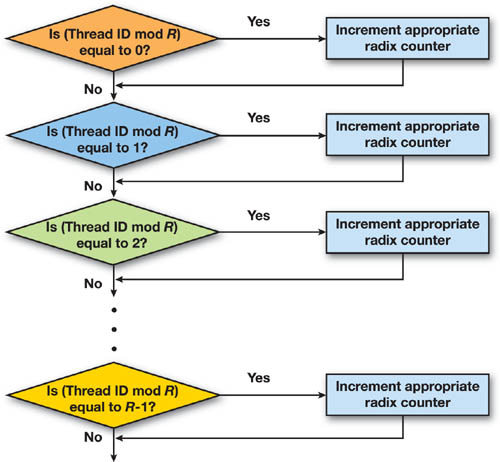Figure 32-11 Flow Chart for Incrementing a Set of Radix Counters That Is Shared by the Threads Within a Thread Group

To maintain the stability of the sort, threads need to write data to the output array in the same order as they appear in the input array. The most straightforward way to achieve this is to have both successive thread blocks and successive threads themselves process successive data segments of the input array.

Therefore, we first assign successive segments of the input array to successive thread blocks, as illustrated in Figure 32-12. Empirical analysis has indicated that using one thread block per multiprocessor performs best. Therefore, because we are targeting the GeForce 8800 GTX with its 16 multiprocessors, we use Num_Blocks = 16 thread blocks; but the extension to a GPU with more multiprocessors is straightforward.

Similarly, within a thread block, as illustrated in Figure 32-13, we assign to successive thread groups successive segments of the section of the input array assigned to the block.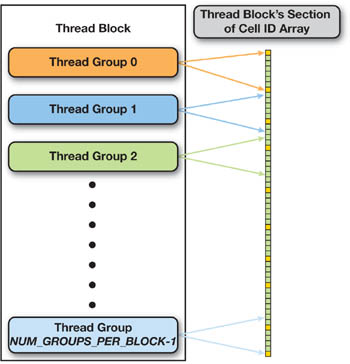Figure 32-13 Mapping Between the Thread Groups of a Thread Block and the Section of the Input Cell ID Array Assigned to This Block

Within a thread group, threads read data in an interleaved way, though, as illustrated in Figure 32-14. This is to make sure that global memory reads are coalesced. To maintain the stability of the sort, we will thus have to serialize the writes to global memory by each thread of a group when it happens in phase 3.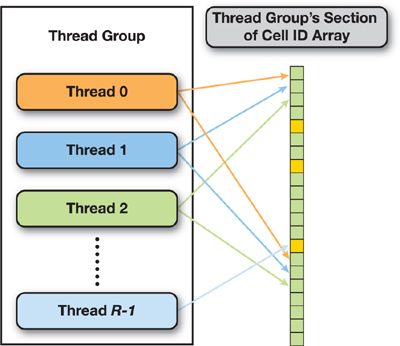Figure 32-14 Mapping Between the Threads of a Thread Group and the Section of the Input Cell ID Array Assigned to This Group

Given this mapping between the threads and the cell ID input array, the index of the first cell ID read by each thread during tabulation is the following: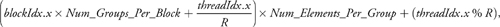where Num_Elements_Per_Group is the size of the data segment assigned to each thread group. Each thread then jumps in increments of R until it reaches the end of its thread group's data segment.

The radix counters are laid out in shared memory first by radix, second by thread block, and third by thread group, as illustrated in Figure 32-15. At the end of the tabulation, we must combine the radix counters of each thread block. Because this requires inter-thread-block synchronization, this can only be done by saving to global memory the counters computed by each block in this phase and calling another kernel; this is therefore left for the next phase.

In phase 3, the input cell ID array will get read by each block in the same way as in phase 1, and each block will output its assigned cell IDs to the right location in the output cell ID array. These locations are determined by offsets in the output cell ID array, and to calculate these offsets, we need the following:

1. For each radix, the prefix sum of each of its radix counters (so, one prefix sum per line of Figure 32-15)
3. The prefix sum of the Num_Radices total sums computed in step 2

The prefix sum from step 3 gives the offsets in the output cell ID array of each section of cell ID with the same radix. The prefix sums from step 1 give the offsets in each of these sections of the subsection where each block will write its assigned cell IDs. In phase 2, we compute steps 1, 2, and part of 3 as detailed now.

The work is broken down among the blocks by having each block take care of Num_Radices_Per_Block = Num_Radices/Num_Blocks radices—so, 16 radices in our case, as illustrated in Figure 32-16.

There are as many radix counters per radix as there are thread groups; in other words, Num_Groups = Num_Blocks x Num_Groups_Per_Block radix counters per radix. So, each block allocates Num_Groups 32-bit values in shared memory. It then iterates through its Num_Radices_Per_Block assigned radices, and for each of them, the following operation is performed by all threads of the block:

1. The Num_Groups counters of the radix are read from global memory to shared memory.
2. The prefix sum of the Num_Groups counters is performed.
3. The Num_Groups counters in global memory are overwritten with the result of the prefix sum.
4. The total sum of the Num_Groups counters is computed by simply adding together the value of the last counter before the prefix sum to its value after the prefix sum.

In step 1, the reads are organized such that threads with consecutive IDs read consecutive counters in memory to get proper memory coalescing.

In step 2, a work-efficient parallel implementation of prefix sum in CUDA is employed, such as the one described in detail in Chapter 39. As explained in this chapter, in order to limit bank conflicts as much as possible, the shared memory array containing the Num_Groups counters needs to be padded appropriately (and therefore more memory must be allocated).

Each block also allocates an array of Num_Radices_Per_Block 32-bit values in shared memory to hold the prefix sum of the Num_Radices_Per_Block total sums computed in step 4. This prefix sum is performed simply by adding the total sum computed at the end of iteration N to element N - 1 of the array.

So at the end of this phase, each thread block has performed a prefix sum on its assigned Num_Radices_Per_Block counters, as illustrated in Figure 32-17, but in order to obtain absolute offsets in the output cell ID array, the total sum of all preceding thread blocks needs to be added to each element of this prefix sum. Because this requires inter-thread-block synchronization, this can only be done by saving to global memory the prefix sum computed by each block in this phase and then calling another kernel; this is therefore left for the next phase.

#### Phase 3: Reordering

The final phase of each pass is used to reorder the input cell ID array based on the radix tabulation performed in phases 1 and 2.

As mentioned at the end of phase 2, each block must first finish the work—partially done in phase 2—of computing the prefix sum of the Num_Radices radix counters in order to get absolute offsets in the output cell ID array. For this, it loads from global memory to shared memory the Num_Blocks prefix sums (each of them being composed of Num_Radices_Per_Block values) computed by each block in phase 2, performs a parallel prefix sum on the last values of each of these prefix sums, and uses it to offset each value of each prefix sum appropriately. The parallel prefix sum performed here is repeated by each block and is inefficient in that it uses only Num_Blocks threads, but it is very fast because it requires few operations.

Next, each block loads its Num_Groups_Per_Block x Num_Radices radix counters from global memory to shared memory (so, one of the Num_Blocks columns of Figure 32-17) and offsets each of them with the appropriate absolute offsets previously computed.

Finally, each thread block reads from global memory the same section of the input cell ID array it read in phase 1, in the same order, and for each cell ID, it does the following:

2. Writes the cell ID to the output cell ID array at the offset indicated by the radix counter

The write and increment operations of steps 2 and 3, respectively, must be serialized for each thread of a half-warp, as illustrated in Figure 32-18; this is necessary both to avoid any shared and global memory hazards between threads of the same half-warp and to maintain the order of cell IDs with the same radix.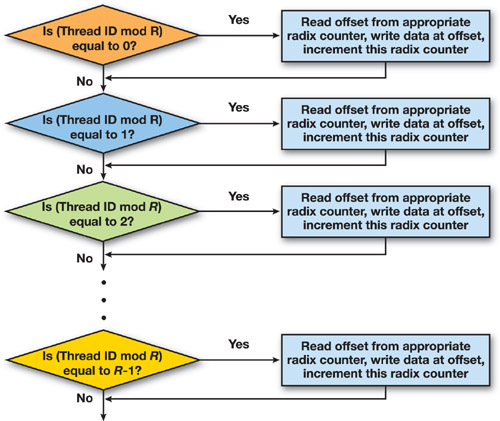Figure 32-18 Increments of Radix Counters in Shared Memory and Writes to Global Memory Need to Be Serialized for Each Thread of a Half-Warp

Step 2 produces extremely scattered and thus uncoalesced writes to global memory, causing a roughly 50 percent performance penalty, but the low pass count of the overall algorithm more than makes up for it.

#### 32.2.4 Creating the Collision Cell List

A collision cell is a cell that requires processing in the narrow phase. In other words, it is a cell that contains more than one object. The list of all the collision cells is created from the sorted cell ID array as follows. The sorted cell ID array is scanned for changes of cell ID, the occurrence of which marks the end of the list of occupants of one cell and the beginning of another. To parallelize this task, we give each thread of each thread block a roughly equal segment of the cell ID array to scan for transitions. Because we want both the beginning and the end of each swath of identical cell IDs to be found by the same thread, each thread scans past the end point of its assigned segment for a final transition and skips its first transition under the assumption that it will be located and recorded by a preceding thread. The only exception is for the first thread of the first thread block, which handles the beginning of the cell ID array and so does not skip its first transition. Also, because we computed the number of valid cells in Section 32.2.2, we can ignore all invalid cell IDs from here on.

The sorted cell ID array is actually scanned twice. The first time, we count the number of objects each collision cell contains and convert them into offsets in the array through a parallel prefix sum in several passes, much like what we have done in phase 2 of the radix sort. The second time, we create entries for each collision cell in a new array. Each collision cell's entry records its start, the number of H occupants, and the number of P occupants, as shown in Figure 32-19. As explained in Section 32.1.3, these cells are also optionally divided into 2 d different lists of noninteracting cells, and the collision processing proceeds with 2 d passes, each of which focuses on one of these lists.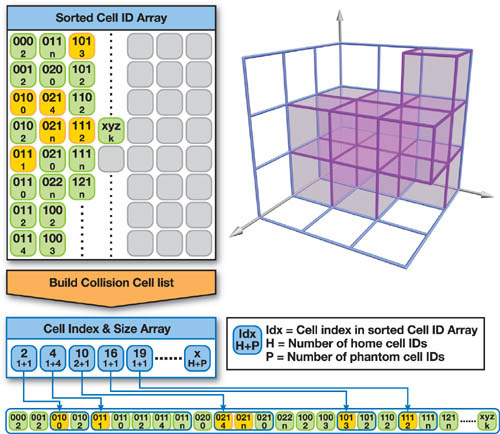Figure 32-19 Scanning the Sorted Cell ID List for Transitions in Order to Locate Collision Cells

#### 32.2.5 Traversing the Collision Cell List

Each collision cell list is then traversed, and collision cells are assigned for processing on a per-thread basis, as illustrated in Figure 32-20. In applications such as molecular dynamics or particle simulation, potential collisions can be processed immediately, allowing the traversal to serve as narrow-phase collision detection. For higher-level simulation, the traversal just records all collisions that pass bounding volume overlap for more detailed processing in a subsequent narrow phase; such simulations are beyond the scope of this chapter.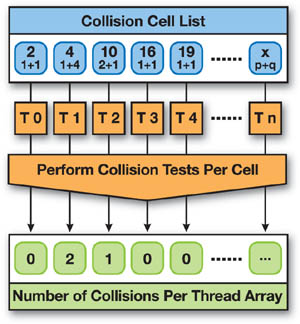Figure 32-20 Thread Layout for Processing Individual Collision Cells on a Per-Thread Basis

Because there is only one thread per collision cell, full utilization of the GPU is achieved only if there are thousands of collision cells to process. This issue becomes more pronounced during Gauss-Seidel physics processing, wherein the collision cells are processed during eight individual passes.

## 32.3 Performance Results

We tested the algorithm with Gauss-Seidel physics on typical configurations of 3,072 to 30,720 dispersed but potentially colliding objects. For the latter, broad phase prunes 450,000,000 potential collisions down to approximately 203,000 on average, and we obtained a frame rate of around 79 frames/sec on a GeForce 8800 GTX. This is approximately 26 times faster than a CPU implementation that was written at the same time as a relative performance benchmark. As illustrated in Figure 32-21, as we decrease object count, frame rate improves dramatically, to a peak of 487 frames/sec with 3,072 objects.

Figure 32-22 illustrates the total amount of time spent in each phase of the algorithm. It is clear that the cell ID array sort and collision cell traversal consume the most time, thus making them the obvious targets for future optimization.

## 32.4 Conclusion

In this chapter, we have shown that CUDA is a great platform for high-speed collision detection. Future work will focus on sharing collision cell processing between multiple threads and on exploring alternative approaches to spatial subdivision.

## 32.5 References

Erleben, Kenny, Joe Sporring, Knud Henriksen, and Henrik Dohlmann. 2005. Physics-Based Animation. Charles River Media. See especially pp. 613–616.

Mirtich, Brian. 1996. "Impulse-Based Dynamic Simulation of Rigid Body Systems." Ph.D. thesis. Department of Computer Science, University of California, Berkeley.

Sedgewick, Robert. 1992. Algorithms in C++. Addison-Wesley. See especially pp. 133–144.

Witkin, A., and D. Baraff. 1997. "An Introduction to Physically Based Modeling: Rigid Body Simulation II—Nonpenetration Constraints." SIGGRAPH 1997 Course 19.

The author would like to thank Samuel Gateau for his many helpful figures and for showing the author his way around Office 2007, and Cyril Zeller for proofreading above and beyond the call of duty.